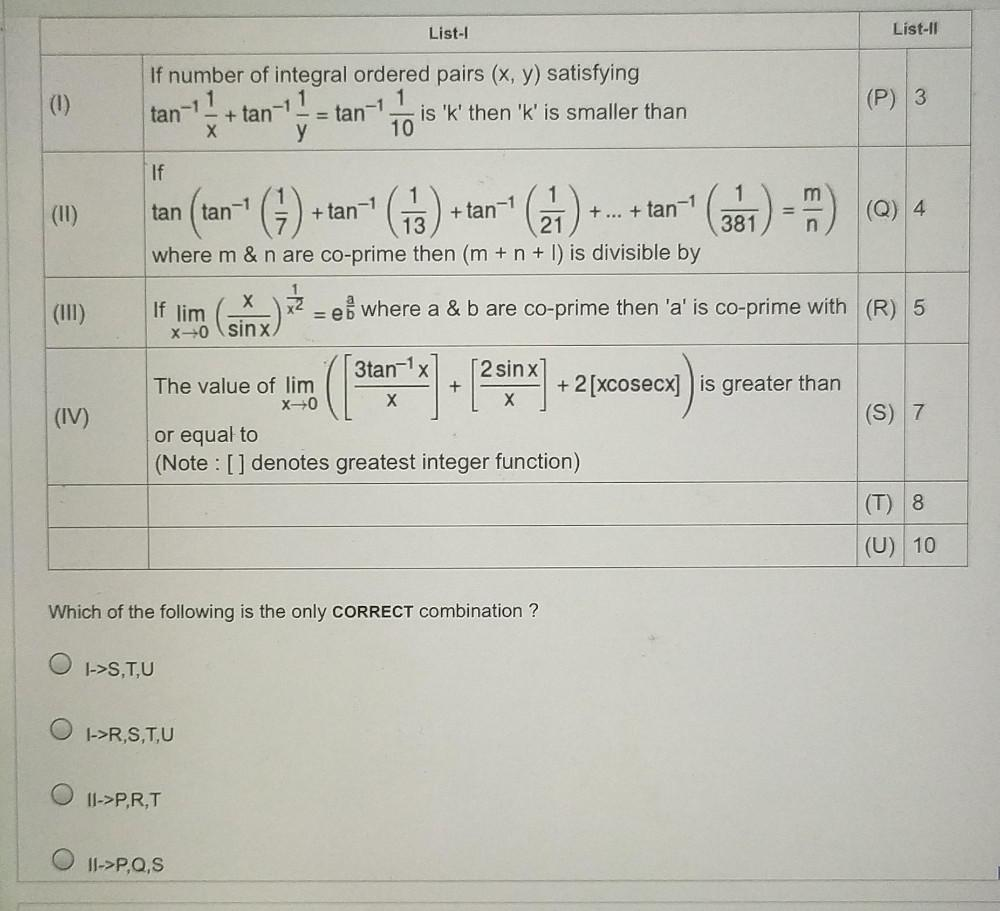Question:

# List-1 List-IT (0) If number of integral ordered pairs (x, y) satisfying 1 + tan-11 - tan-1 is 'k' then 'k' is smaller than y 10List-1 List-IT (0) If number of integral ordered pairs (x, y) satisfying 1 + tan-11 - tan-1 is 'k' then 'k' is smaller than y 10 (P) 3 tan- (11) G () + tan-1 (63) + G + tan-1 + ... + tan-1 ( - 21 X (III) If 1 1 1 tan (tan-1 13 (Q) 4 381 where m & n are co-prime then (m + n + 1) is divisible by If lim x2 x2 = ei where a & b are co-prime then 'a' is co-prime with (R) 5 x-o sinx 3tan-1 X 2 sin x) The value of lim + + 2[xcosecx] is greater than X=0 X X (S) 7 or equal to (Note : [ ] denotes greatest integer function) (I 1) (IV) (T) 8 (U) 10 Which of the following is the only CORRECT combination ? O O 1->S,T,U O ->R,S,T,U 11->P,R,T O 11->P,Q,S